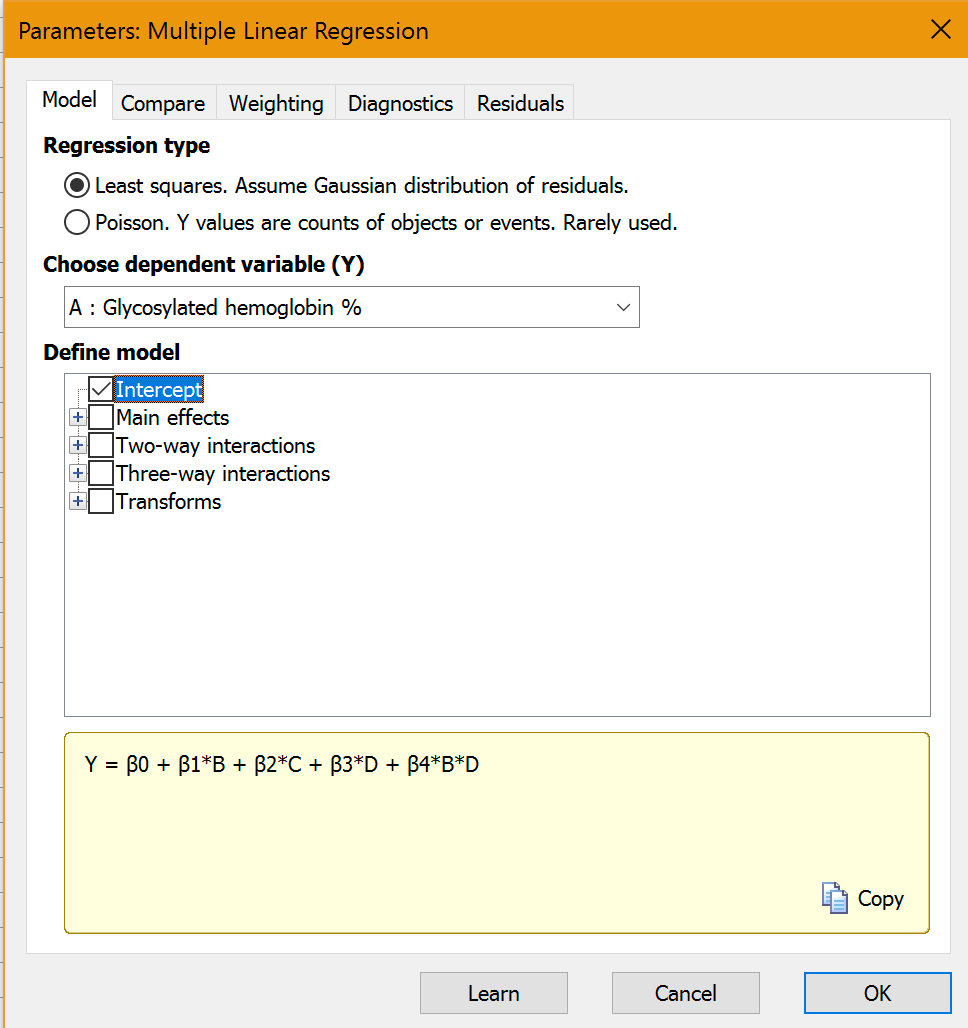## Please enable JavaScript to view this site.

 Multiple regression

New kind of table

Prism 8 offers a new kind of data table, multiple variables. It is arranged like most statistical programs. Each row represents a different subject or experiment or unit. Each column is for a different variable.

Multiple linear regressionThe word "multiple" means that there are several independent (X) variables. Multiple linear regression fits an equation that predicts Y based on a linear combination of X variables. This is a standard analysis that you can read about in many books. Options:

If the Y values are numbers of objects or events actually counted, Prism can do Poisson regression. If Y is a continuous variable, Prism does multiple linear regression.

Include (or not) the intercept, two-way interactions between selected variables, and three-way interactions between selected variables.

Include transforms of the parameters in the model.

Weight all the points equally or weight by 1/Y2 or some other weighting functions.  Weighting is useful when variability is larger for points with larger Y values than for points with smaller Y values.

Fit two alternative models and compare the goodness of  fits.

Graph actual vs. predicted (from the model) Y values.

Graph the residuals in four ways.

Test for normality of the residuals, and for multicollinearity of the parameters.

Multiple logistic regression

New in version 8.3.0, Prism can now perform Multiple logistic regression. As with multiple linear regression, the word "multiple" here means that there are several independent (X) variables, or predictors. Multiple logistic regression allows you to fit a model to your data when your outcome variable (Y) is binary: yes or no, 1 or 0, alive or dead, etc.

New analysis to extract and rearrange data

A new "Extract and rearrange" analysis lets you extract data from a portion of a multiple variable table and use it to create another kind of table. For example, you can move two columns in to a new XY table. Or put data from one column into a Column table using another column to define which data set each value goes into. Or cross-tabulate onto a chi-square table.

New analysis to select and transform and combine

Another analysis (Select and Transform) creates a new multiple variable table from an existing one. For example, you could select rows 1-100. Or if one of the columns is age, you could select only the rows of people older than 75.  This analysis can also create new variables by transforming variables or by combining variables (for example take the ratio of two columns).